# simulate

Monte Carlo simulation of vector autoregression (VAR) model

## Syntax

``Y = simulate(Mdl,numobs)``
``Y = simulate(Mdl,numobs,Name=Value)``
``````[Y,E] = simulate(___)``````
``Tbl = simulate(Mdl,numobs,Presample=Presample)``
``Tbl = simulate(Mdl,numobs,Presample=Presample,Name=Value)``
``Tbl = simulate(Mdl,numobs,InSample=InSample,ResponseVariables=ResponseVariables)``
``Tbl = simulate(Mdl,numobs,InSample=InSample,ResponseVariables=ResponseVariables,Presample=Presample)``
``Tbl = simulate(___,Name=Value)``

## Description

### Conditional and Unconditional Simulation for Numeric Arrays

example

````Y = simulate(Mdl,numobs)` returns the numeric array `Y` containing a random `numobs`-period path of multivariate response series from performing an unconditional simulation of the fully specified VAR(p) model `Mdl`.```

example

````Y = simulate(Mdl,numobs,Name=Value)` uses additional options specified by one or more name-value arguments. `simulate` returns numeric arrays when all optional input data are numeric arrays. For example, `simulate(Mdl,100,NumPaths=1000,Y0=PS)` returns a numeric array of 1000, 100-period simulated response paths from `Mdl` and specifies the numeric array of presample response data `PS`.To produce a conditional simulation, specify response data in the simulation horizon by using the `YF` name-value argument.```

example

``````[Y,E] = simulate(___)``` also returns the numeric array containing the simulated multivariate model innovations series `E` corresponding to the simulated responses `Y`, using any input argument combination in the previous syntaxes.```

### Unconditional Simulation for Tables and Timetables

example

````Tbl = simulate(Mdl,numobs,Presample=Presample)` returns the table or timetable `Tbl` containing the random multivariate response and innovations variables, which results from the unconditional simulation of the response series in the model `Mdl`. `simulate` uses the table or timetable of presample data `Presample` to initialize the response series. (since R2022b)`simulate` selects the variables in `Mdl.SeriesNames` to simulate, or it selects all variables in `Presample`. To select different response variables in `Tbl` to simulate, use the `PresampleResponseVariables` name-value argument.```

example

````Tbl = simulate(Mdl,numobs,Presample=Presample,Name=Value)` uses additional options specified by one or more name-value arguments. For example, ```simulate(Mdl,100,Presample=PSTbl,PresampleResponseVariables=["GDP" "CPI"])``` returns a timetable of variables containing 100-period simulated response and innovations series from `Mdl`, initialized by the data in the `GDP` and `CPI` variables of the timetable of presample data in `PSTbl`. (since R2022b)```

### Conditional Simulation for Tables and Timetables

example

````Tbl = simulate(Mdl,numobs,InSample=InSample,ResponseVariables=ResponseVariables)` returns the table or timetable `Tbl` containing the random multivariate response and innovations variables, which results from the conditional simulation of the response series in the model `Mdl`. `InSample` is a table or timetable of response or predictor data in the simulation horizon that `simulate` uses to perform the conditional simulation and `ResponseVariables` specifies the response variables in `InSample`. (since R2022b)```

example

````Tbl = simulate(Mdl,numobs,InSample=InSample,ResponseVariables=ResponseVariables,Presample=Presample)` uses the presample data in the table or timetable `Presample` to initialize the model. (since R2022b)```

example

````Tbl = simulate(___,Name=Value)` uses additional options specified by one or more name-value arguments, using any input argument combination in the previous two syntaxes. (since R2022b)```

## Examples

collapse all

Fit a VAR(4) model to the consumer price index (CPI) and unemployment rate data. Then, simulate a vector of responses from the estimated model.

Load the `Data_USEconModel` data set.

`load Data_USEconModel`

Plot the two series on separate plots.

```figure plot(DataTimeTable.Time,DataTimeTable.CPIAUCSL); title("Consumer Price Index") ylabel("Index") xlabel("Date")``````figure plot(DataTimeTable.Time,DataTimeTable.UNRATE); title("Unemployment Rate") ylabel("Percent") xlabel("Date")```Stabilize the CPI by converting it to a series of growth rates. Synchronize the two series by removing the first observation from the unemployment rate series. Create a new data set containing the transformed variables, and do not include any rows containing at least one missing observation.

```rcpi = price2ret(DataTimeTable.CPIAUCSL); unrate = DataTimeTable.UNRATE(2:end); dates = DataTimeTable.Time(2:end); Data = array2timetable([rcpi unrate],RowTimes=dates, ... VariableNames=["RCPI" "UNRATE"]); Data = rmmissing(Data);```

Create a default VAR(4) model by using the shorthand syntax.

```Mdl = varm(2,4); Mdl.SeriesNames = Data.Properties.VariableNames;```

Estimate the model using the entire data set.

`EstMdl = estimate(Mdl,Data.Variables);`

`EstMdl` is a fully specified, estimated `varm` model object.

Simulate a response series path from the estimated model with length equal to the path in the data.

```rng(1); % For reproducibility numobs = height(Data); Y = simulate(EstMdl,numobs);```

`Y` is a 245-by-2 matrix of simulated responses. The first and second columns contain the simulated CPI growth rate and unemployment rate, respectively.

Plot the simulated and true responses.

```figure plot(Data.Time,Y(:,1)); hold on plot(Data.Time,Data.RCPI) title("CPI Growth Rate"); ylabel("Growth Rate") xlabel("Date") legend("Simulation","Observed") hold off```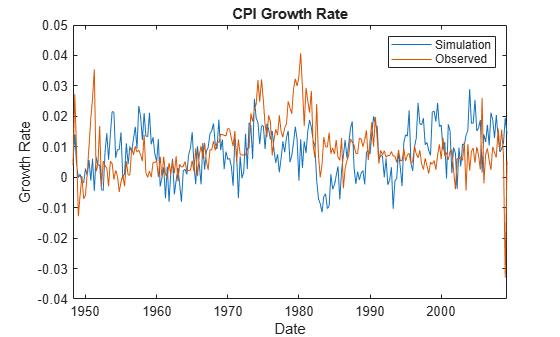```figure plot(Data.Time,Y(:,2)); hold on plot(Data.Time,Data.UNRATE) ylabel("Percent") xlabel("Date") title("Unemployment Rate") legend("Simulation","Observed") hold off```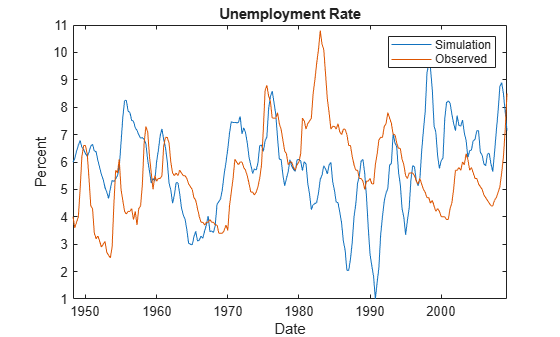Illustrate the relationship between `simulate` and `filter` by estimating a 4-D VAR(2) model of the four response series in Johansen's Danish data set. Simulate a single path of responses using the fitted model and the historical data as initial values, and then filter a random set of Gaussian disturbances through the estimated model using the same presample responses.

`load Data_JDanish`

For details on the variables, enter `Description`.

Create a default 4-D VAR(2) model.

```Mdl = varm(4,2); Mdl.SeriesNames = DataTimeTable.Properties.VariableNames;```

Estimate the VAR(2) model using the entire data set.

`EstMdl = estimate(Mdl,DataTimeTable.Variables);`

When reproducing the results of `simulate` and `filter`:

• Set the same random number seed using `rng`.

• Specify the same presample response data using the `Y0` name-value argument.

Set the default random seed. Simulate 100 observations by passing the estimated model to `simulate`. Specify the entire data set as the presample.

```rng("default") YSim = simulate(EstMdl,100,Y0=DataTimeTable.Variables);```

`YSim` is a 100-by-4 matrix of simulated responses. Columns correspond to the columns of the variables in `Data`.

Set the default random seed. Simulate 4 series of 100 observations from the standard Gaussian distribution.

```rng("default") Z = randn(100,4);```

Filter the Gaussian values through the estimated model. Specify the entire data set as the presample.

`YFilter = filter(EstMdl,Z,Y0=DataTimeTable.Variables);`

`YFilter` is a 100-by-4 matrix of simulated responses. Columns correspond to the columns of the variables in the data `Data`. Before filtering the disturbances, `filter` scales `Z` by the lower triangular Cholesky factor of the model covariance in `EstMdl.Covariance`.

Compare the resulting responses between `filter` and `simulate`.

`(YSim - YFilter)'*(YSim - YFilter)`
```ans = 4×4 0 0 0 0 0 0 0 0 0 0 0 0 0 0 0 0 ```

The results are identical.

Load Johansen's Danish economic data. Remove all missing observations.

```load Data_JDanish Data = rmmissing(Data); T = height(Data);```

For details on the variables, enter `Description`.

Create a default 4-D VAR(2) model.

`Mdl = varm(4,2);`

Estimate the VAR(2) model using the entire data set.

`EstMdl = estimate(Mdl,Data);`

When reproducing the results of `simulate` and `filter`:

• Set the same random number seed using `rng`.

• Specify the same presample response data using the `Y0` name-value argument.

Simulate 100 paths of `T – EstMdl.P`, the effective sample size, responses, and corresponding innovations by passing the estimated model to `simulate`. Specify the same matrix of presample as the presample used in estimation (the earliest `Mdl.P` observations, by default).

```rng("default") p = Mdl.P; numobs = T - p; PS = Data(1:p,:); [YSim,ESim] = simulate(EstMdl,numobs,NumPaths=100,Y0=PS); size(YSim)```
```ans = 1×3 53 4 100 ```

`YSim` and `ESim` are 53-by-4-by-1000 numeric arrays of simulated responses and innovations, respectively. Each row corresponds to a period in the simulation horizon, each column corresponds to the variable in `EstMdl.SeriesNames`, and pages are separate, independently simulated paths.

Plot each simulated response and innovations variable with their observations.

```figure InSample = Data((p+1):end,:); tiledlayout(2,2) for j = 1:numel(EstMdl.SeriesNames) nexttile h1 = plot(squeeze(YSim(:,j,:)),Color=[0.8 0.8 0.8]); hold on h2 = plot(InSample(:,j),Color="k",LineWidth=2); hold off title(series(j)) legend([h1(1) h2],["Simulated" "Observed"]) end```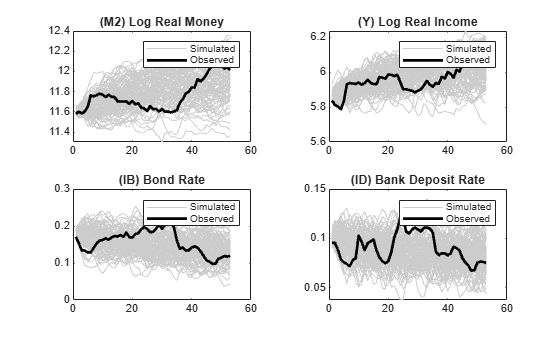```E = infer(EstMdl,InSample,Y0=PS); figure tiledlayout(2,2) for j = 1:numel(EstMdl.SeriesNames) nexttile h1 = plot(squeeze(ESim(:,j,:)),Color=[0.8 0.8 0.8]); hold on h2 = plot(E(:,j),Color="k",LineWidth=2); hold off title("Innovations: " + EstMdl.SeriesNames{j}) legend([h1(1) h2],["Simulated" "Observed"]) end```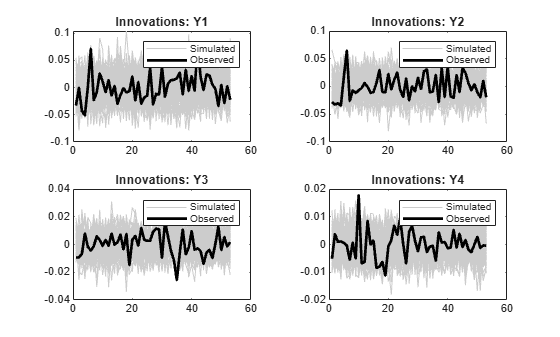Since R2022b

Fit a VAR(4) model to the consumer price index (CPI) and unemployment rate data. Then, perform an unconditional simulation of the estimated model and return the simulated responses and corresponding innovations in a timetable. This example is based on Return Response Series in Matrix from Unconditional Simulation.

Load the `Data_USEconModel` data set. Compute the CPI growth rate. Because the growth rate calculation consumes the earliest observation, include the rate variable in the timetable by prepending the series with `NaN`.

```load Data_USEconModel DataTimeTable.RCPI = [NaN; price2ret(DataTimeTable.CPIAUCSL)]; T = height(DataTimeTable)```
```T = 249 ```

Prepare Timetable for Estimation

When you plan to supply a timetable directly to estimate, you must ensure it has all the following characteristics:

• All selected response variables are numeric and do not contain any missing values.

• The timestamps in the `Time` variable are regular, and they are ascending or descending.

Remove all missing values from the table, relative to the CPI rate (`RCPI`) and unemployment rate (`UNRATE`) series.

```varnames = ["RCPI" "UNRATE"]; DTT = rmmissing(DataTimeTable,DataVariables=varnames); T = height(DTT)```
```T = 245 ```

`rmmissing` removes the four initial missing observations from the `DataTimeTable` to create a sub-table `DTT`. The variables `RCPI` and `UNRATE` of `DTT` do not have any missing observations.

Determine whether the sampling timestamps have a regular frequency and are sorted.

`areTimestampsRegular = isregular(DTT,"quarters")`
```areTimestampsRegular = logical 0 ```
`areTimestampsSorted = issorted(DTT.Time)`
```areTimestampsSorted = logical 1 ```

`areTimestampsRegular = 0` indicates that the timestamps of `DTT` are irregular. `areTimestampsSorted = 1` indicates that the timestamps are sorted. Macroeconomic series in this example are timestamped at the end of the month. This quality induces an irregularly measured series.

Remedy the time irregularity by shifting all dates to the first day of the quarter.

```dt = DTT.Time; dt = dateshift(dt,"start","quarter"); DTT.Time = dt; areTimestampsRegular = isregular(DTT,"quarters")```
```areTimestampsRegular = logical 1 ```

`DTT` is regular with respect to time.

Create Model Template for Estimation

Create a default VAR(4) model by using the shorthand syntax. Specify the response variable names.

```Mdl = varm(2,4); Mdl.SeriesNames = varnames;```

Fit Model to Data

Estimate the model. Pass the entire timetable `DTT`. By default, `estimate` selects the response variables in `Mdl.SeriesNames` to fit to the model. Alternatively, you can use the `ResponseVariables` name-value argument.

```EstMdl = estimate(Mdl,DTT); p = EstMdl.P```
```p = 4 ```

Perform Unconditional Simulation of Estimated Model

Simulate a response and innovations path from the estimated model and return the simulated series as variables in a timetable. `simulate` requires information for the output timetable, such as variable names, sampling times for the simulation horizon, and sampling frequency. Therefore, supply a presample of the earliest `p` = 4 observations of the data `DTT`, from which `simulate` infers the required timetable information. Specify a simulation horizon of `numobs – p`.

```rng(1) % For reproducibility PSTbl = DTT(1:p,:); numobs = T - p; Tbl = simulate(EstMdl,T,Presample=PSTbl); size(Tbl)```
```ans = 1×2 245 4 ```
`PSTbl`
```PSTbl=4×15 timetable Time COE CPIAUCSL FEDFUNDS GCE GDP GDPDEF GPDI GS10 HOANBS M1SL M2SL PCEC TB3MS UNRATE RCPI _____ _____ ________ ________ ____ _____ ______ ____ ____ ______ ____ ____ _____ _____ ______ _________ Q1-48 137.9 23.5 NaN 37.6 260.4 16.111 45 NaN 55.036 NaN NaN 170.5 1 4 0.0038371 Q2-48 139.6 24.15 NaN 39.7 267.3 16.254 48.1 NaN 55.007 NaN NaN 174.3 1 3.6 0.027284 Q3-48 144.5 24.36 NaN 41.4 273.9 16.556 50.2 NaN 55.398 NaN NaN 177.2 1.09 3.8 0.0086581 Q4-48 145.9 24.05 NaN 43.5 275.2 16.597 49.1 NaN 54.885 NaN NaN 178.1 1.16 4 -0.012807 ```
`head(Tbl)`
``` Time RCPI_Responses UNRATE_Responses RCPI_Innovations UNRATE_Innovations _____ ______________ ________________ ________________ __________________ Q1-49 0.0037294 4.6036 -0.0038547 0.25039 Q2-49 0.0064827 5.0083 0.0070154 0.027504 Q3-49 -0.0073358 5.4981 -0.0045047 0.25199 Q4-49 -0.0057328 5.7007 -0.0065904 0.10593 Q1-50 -0.0060454 5.8687 -0.005022 0.13824 Q2-50 -0.0084475 5.5758 -0.0034013 -0.26192 Q3-50 -0.0067066 5.4129 -0.0033182 0.13055 Q4-50 -0.0020759 5.2191 0.0010595 0.11135 ```

`Tbl` is a 241-by-4 matrix of simulated responses and innovations. `RCPI_Responses` is the simulated path of the CPI growth rate and `RCPI_Innovations` is the corresponding innovations series, and the variables associated with the unemployment rate are similar. The timestamps of `Tbl` follow directly from the timestamps of `PSTbl`, and they have the same sampling frequency.

Since R2022b

Estimate a VAR(4) model of the consumer price index (CPI), the unemployment rate, and the gross domestic product (GDP). Include a linear regression component containing the current and the last four quarters of government consumption expenditures and investment. Simulate multiple paths from the estimated model.

Load the `Data_USEconModel` data set. Compute the real GDP.

```load Data_USEconModel DataTimeTable.RGDP = DataTimeTable.GDP./DataTimeTable.GDPDEF*100;```

Plot all variables on separate plots.

```figure tiledlayout(2,2) nexttile plot(DataTimeTable.Time,DataTimeTable.CPIAUCSL); ylabel("Index") title("Consumer Price Index") nexttile plot(DataTimeTable.Time,DataTimeTable.UNRATE); ylabel("Percent") title("Unemployment Rate") nexttile plot(DataTimeTable.Time,DataTimeTable.RGDP); ylabel("Output") title("Real Gross Domestic Product") nexttile plot(DataTimeTable.Time,DataTimeTable.GCE); ylabel("Billions of \$") title("Government Expenditures")```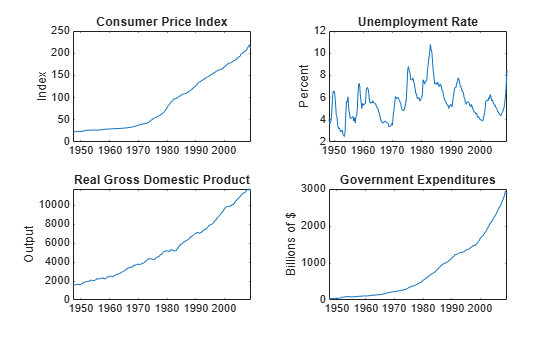Stabilize the CPI, GDP, and GCE by converting each to a series of growth rates. Synchronize the unemployment rate series with the others by removing its first observation.

```varnames = ["CPIAUCSL" "RGDP" "GCE"]; DTT = varfun(@price2ret,DataTimeTable,InputVariables=varnames); DTT.Properties.VariableNames = varnames; DTT.UNRATE = DataTimeTable.UNRATE(2:end);```

Make the time base regular.

```dt = DTT.Time; dt = dateshift(dt,"start","quarter"); DTT.Time = dt;```

Expand the GCE rate series to a matrix that includes the first lagged series through the fourth lag series.

```RGCELags = lagmatrix(DTT,1:4,DataVariables="GCE"); DTT = [DTT RGCELags]; DTT = rmmissing(DTT);```

Create separate presample and estimation sample data sets. The presample contains the earliest `p` = `4` observations, and the estimation sample contains the rest of the data.

```p = 4; PS = DTT(1:p,:); InSample = DTT((p+1):end,:); respnames = ["CPIAUCSL" "UNRATE" "RGDP"]; idx = endsWith(InSample.Properties.VariableNames,"GCE"); prednames = InSample.Properties.VariableNames(idx);```

Create a default VAR(4) model by using the shorthand syntax. Specify the response variable names.

```Mdl = varm(3,p); Mdl.SeriesNames = respnames;```

Estimate the model using the entire sample. Specify the GCE and its lags as exogenous predictor data for the regression component.

`EstMdl = estimate(Mdl,InSample,Presample=PS,PredictorVariables=prednames);`

Generate 100 random response and innovations paths from the estimated model by performing an unconditional simulation. Specify that the length of the paths is the same as the length of the estimation sample period. Supply the presample and estimation sample data.

```rng(1) % For reproducibility numpaths = 100; numobs = height(InSample); Tbl = simulate(EstMdl,numobs,NumPaths=numpaths, ... Presample=PS,InSample=InSample,PredictorVariables=prednames); size(Tbl)```
```ans = 1×2 240 14 ```
`head(Tbl)`
``` Time CPIAUCSL RGDP GCE UNRATE Lag1GCE Lag2GCE Lag3GCE Lag4GCE CPIAUCSL_Responses UNRATE_Responses RGDP_Responses CPIAUCSL_Innovations UNRATE_Innovations RGDP_Innovations _____ __________ __________ __________ ______ __________ __________ __________ __________ __________________ ________________ ______________ ____________________ __________________ ________________ Q1-49 0.00041815 -0.0031645 0.036603 6.2 0.047147 0.04948 0.04193 0.054347 1x100 double 1x100 double 1x100 double 1x100 double 1x100 double 1x100 double Q2-49 -0.0071324 0.011385 -0.0021164 6.6 0.036603 0.047147 0.04948 0.04193 1x100 double 1x100 double 1x100 double 1x100 double 1x100 double 1x100 double Q3-49 -0.0059122 -0.010366 -0.012793 6.6 -0.0021164 0.036603 0.047147 0.04948 1x100 double 1x100 double 1x100 double 1x100 double 1x100 double 1x100 double Q4-49 0.0012698 0.040091 -0.021693 6.3 -0.012793 -0.0021164 0.036603 0.047147 1x100 double 1x100 double 1x100 double 1x100 double 1x100 double 1x100 double Q1-50 0.010101 0.029649 0.010905 5.4 -0.021693 -0.012793 -0.0021164 0.036603 1x100 double 1x100 double 1x100 double 1x100 double 1x100 double 1x100 double Q2-50 0.01908 0.03844 -0.0043478 4.4 0.010905 -0.021693 -0.012793 -0.0021164 1x100 double 1x100 double 1x100 double 1x100 double 1x100 double 1x100 double Q3-50 0.025954 0.017994 0.075508 4.3 -0.0043478 0.010905 -0.021693 -0.012793 1x100 double 1x100 double 1x100 double 1x100 double 1x100 double 1x100 double Q4-50 0.035395 0.01197 0.14807 3.4 0.075508 -0.0043478 0.010905 -0.021693 1x100 double 1x100 double 1x100 double 1x100 double 1x100 double 1x100 double ```

`Tbl` is a 240-by-14 timetable of estimation sample data, simulated responses (denoted `responseName``_Responses`), and corresponding innovations (denoted `responseName``_Innovations`). The simulated response and innovations variables are 240-by-100 matrices, where each row is a period in the estimation sample and each column is a separate, independently generated path.

For each time in the estimation sample, compute the mean vector of the simulated responses among all paths.

```idx = endsWith(Tbl.Properties.VariableNames,"_Responses"); simrespnames = Tbl.Properties.VariableNames(idx); MeanSim = varfun(@(x)mean(x,2),Tbl,InputVariables=simrespnames);```

`MeanSim` is a 240-by-3 timetable containing the average of the simulated responses at each time point.

Plot the simulated responses, their averages, and the data.

```figure tiledlayout(2,2) for j = 1:Mdl.NumSeries nexttile plot(Tbl.Time,Tbl{:,simrespnames(j)},Color=[0.8,0.8,0.8]) title(Mdl.SeriesNames{j}); hold on h1 = plot(Tbl.Time,Tbl{:,respnames(j)}); h2 = plot(Tbl.Time,MeanSim{:,"Fun_"+simrespnames(j)}); hold off end hl = legend([h1 h2],"Data","Mean"); hl.Position = [0.6 0.25 hl.Position(3:4)];```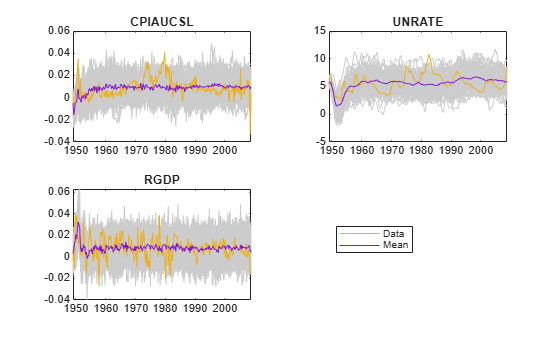Since R2022b

Perform a conditional simulation of the VAR model in Return Timetable of Responses and Innovations from Unconditional Simulation, in which economists hypothesize that the unemployment rate is 6% for 15 quarters after the end of the sampling period (from Q2 of 2009 through Q4 of 2012).

Load the `Data_USEconModel` data set. Compute the CPI growth rate. Because the growth rate calculation consumes the earliest observation, include the rate variable in the timetable by prepending the series with `NaN`.

```load Data_USEconModel DataTimeTable.RCPI = [NaN; price2ret(DataTimeTable.CPIAUCSL)];```

Prepare Timetable for Estimation

Remove all missing values from the table, relative to the CPI rate (`RCPI`) and unemployment rate (`UNRATE`) series.

```varnames = ["RCPI" "UNRATE"]; DTT = rmmissing(DataTimeTable,DataVariables=varnames);```

Remedy the time irregularity by shifting all dates to the first day of the quarter.

```dt = DTT.Time; dt = dateshift(dt,"start","quarter"); DTT.Time = dt;```

Create Model Template for Estimation

Create a default VAR(4) model by using the shorthand syntax. Specify the response variable names.

```p = 4; Mdl = varm(2,p); Mdl.SeriesNames = varnames;```

Fit Model to Data

Estimate the model. Pass the entire timetable `DTT`. By default, `estimate` selects the response variables in `Mdl.SeriesNames` to fit to the model. Alternatively, you can use the `ResponseVariables` name-value argument.

`EstMdl = estimate(Mdl,DTT);`

Prepare for Conditional Simulation of Estimated Model

Suppose economists hypothesize that the unemployment rate will be at 6% for the next 15 quarters.

Create a timetable with the following qualities:

• The timestamps are regular with respect to the estimation sample timestamps and they are ordered from Q2 of 2009 through Q4 of 2012.

• The variable `RCPI` (and, consequently, all other variables in `DTT`) is a 15-by-1 vector of `NaN` values.

• The variable `UNRATE` is a 15-by-1 vector, where each element is 6.

```numobs = 15; shdt = DTT.Time(end) + calquarters(1:numobs); DTTCondSim = retime(DTT,shdt,"fillwithmissing"); DTTCondSim.UNRATE = ones(numobs,1)*6;```

`DTTCondSim` is a 15-by-15 timetable that follows directly, in time, from `DTT`, and both timetables have the same variables. All variables in `DTTCondSim` contain `NaN` values, except for `UNRATE`, which is a vector composed of the value 6.

Perform Conditional Simulation of Estimated Model

Simulate the CPI growth rate given the hypothesis by supplying the conditioning data `DTTCondSim` and specifying the response variable names. Generate 1000 paths. Because the simulation horizon is beyond the estimation sample data, supply the estimation sample as a presample to initialize the model.

```rng(1) % For reproducibility Tbl = simulate(EstMdl,numobs,NumPaths=1000, ... InSample=DTTCondSim,ResponseVariables=EstMdl.SeriesNames, ... Presample=DTT,PresampleResponseVariables=EstMdl.SeriesNames); size(Tbl)```
```ans = 1×2 15 19 ```
```idx = endsWith(Tbl.Properties.VariableNames,["_Responses" "_Innovations"]); head(Tbl(:,idx))```
``` Time RCPI_Responses UNRATE_Responses RCPI_Innovations UNRATE_Innovations _____ ______________ ________________ ________________ __________________ Q2-09 1x1000 double 1x1000 double 1x1000 double 1x1000 double Q3-09 1x1000 double 1x1000 double 1x1000 double 1x1000 double Q4-09 1x1000 double 1x1000 double 1x1000 double 1x1000 double Q1-10 1x1000 double 1x1000 double 1x1000 double 1x1000 double Q2-10 1x1000 double 1x1000 double 1x1000 double 1x1000 double Q3-10 1x1000 double 1x1000 double 1x1000 double 1x1000 double Q4-10 1x1000 double 1x1000 double 1x1000 double 1x1000 double Q1-11 1x1000 double 1x1000 double 1x1000 double 1x1000 double ```

`Tbl` is a 15-by-19 matrix of simulated responses and innovations of `RCPI` given `UNRATE` is 6% for the next 15 quarters. `RCPI_Responses` contains the simulated paths of the CPI growth rate and `RCPI_Innovations` contains the corresponding innovations series. `UNRATE_Responses` is a 15-by-1000 matrix composed of the value 6. All other variables in `Tbl` are the variables and their values in `DTTCondSim`.

Plot the simulated values of the CPI growth rate and their mean with the final few values of the estimation sample data.

```MeanRCPISim = mean(Tbl.RCPI_Responses,2); figure h1 = plot(DTT.Time((end-30):end),DTT.RCPI((end-30):end)); hold on h2 = plot(Tbl.Time,Tbl.RCPI_Responses,Color=[0.8 0.8 0.8]); h3 = plot(Tbl.Time,MeanRCPISim,Color="k",LineWidth=2); xline(Tbl.Time(1),"r--",LineWidth=2) hold off title(EstMdl.SeriesNames) legend([h1 h2(1) h3],["Estimation data" "Simulated paths" "Simulation mean"], ... Location="best")```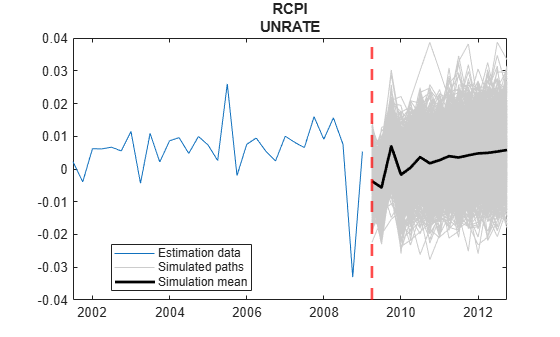## Input Arguments

collapse all

VAR model, specified as a `varm` model object created by `varm` or `estimate`. `Mdl` must be fully specified.

Number of random observations to generate per output path, specified as a positive integer. The output arguments `Y` and `E`, or `Tbl`, have `numobs` rows.

Data Types: `double`

Since R2022b

Presample data that provides initial values for the model `Mdl`, specified as a table or timetable with `numprevars` variables and `numpreobs` rows. The following situations describe when to use `Presample`:

• `Presample` is required when `simulate` performs an unconditional simulation, which occurs under one of the following conditions:

• You do not supply data in the simulation horizon (that is, you do not use the `InSample` name-value argument).

• You specify only predictor data for the model regression component in the simulation horizon using the `InSample` and `PredictorVariables` name-value arguments, but you do not select any response variables from `InSample`.

• `Presample` is optional when `simulate` performs a conditional simulation, that is, when you supply response data in the simulation horizon, on which to condition the simulated responses, by using the `InSample` and `ResponseVariables` name-value arguments. By default, `simulate` sets any necessary presample observations.

• For stationary VAR processes without regression components, `simulate` sets presample observations to the unconditional mean $\mu ={\Phi }^{-1}\left(L\right)c.$

• For nonstationary processes or models that contain a regression component, `simulate` sets presample observations to zero.

Regardless of the situation, `simulate` returns the simulated variables in the output table or timetable `Tbl`, which is commensurate with `Presample`.

Each row is a presample observation, and measurements in each row, among all paths, occur simultaneously. `numpreobs` must be at least `Mdl.P`. If you supply more rows than necessary, `simulate` uses the latest `Mdl.P` observations only.

Each variable is a `numpreobs`-by-`numprepaths` numeric matrix. Variables are associated with response series in `Mdl.SeriesNames`. To control presample variable selection, see the optional `PresampleResponseVariables` name-value argument.

For each variable, columns are separate, independent paths.

• If variables are vectors, `simulate` applies them to each respective path to initialize the model for the simulation. Therefore, all respective response paths derive from common initial conditions.

• Otherwise, for each variable `ResponseK` and each path `j`, `simulate` applies `Presample.ResponseK(:,j)` to produce `Tbl.ResponseK``(:,j)`. Variables must have at least `numpaths` columns, and `simulate` uses only the first `numpaths` columns.

If `Presample` is a timetable, all the following conditions must be true:

• `Presample` must represent a sample with a regular datetime time step (see `isregular`).

• The inputs `InSample` and `Presample` must be consistent in time such that `Presample` immediately precedes `InSample` with respect to the sampling frequency and order.

• The datetime vector of sample timestamps `Presample.Time` must be ascending or descending.

If `Presample` is a table, the last row contains the latest presample observation.

Since R2022b

Future time series response or predictor data, specified as a table or timetable. `InSample` contains `numvars` variables, including `numseries` response variables yt or `numpreds` predictor variables xt for the model regression component. You can specify `InSample` only when other data inputs are tables or timetables.

Use `InSample` in the following situations:

• Perform conditional simulation. You must also supply the response variable names in `InSample` by using the `ResponseVariables` name-value argument.

• Supply future predictor data for either unconditional or conditional simulation. To supply predictor data, you must specify predictor variable names in `InSample` by using the `PredictorVariables` name-value argument. Otherwise, `simulate` ignores the model regression component.

`simulate` returns the simulated variables in the output table or timetable `Tbl`, which is commensurate with `InSample`.

Each row corresponds to an observation in the simulation horizon, the first row is the earliest observation, and measurements in each row, among all paths, occur simultaneously. `InSample` must have at least `numobs` rows to cover the simulation horizon. If you supply more rows than necessary, `simulate` uses only the first `numobs` rows.

Each response variable is a numeric matrix with `numpaths` columns. For each response variable `K`, columns are separate, independent paths. Specifically, path `j` of response variable `ResponseK` captures the state, or knowledge, of `ResponseK` as it evolves from the presample past (for example, `Presample.ResponseK`) into the future. For each selected response variable `ResponseK`:

• If `InSample.ResponseK` is a vector, `simulate` applies to each of the `numpaths` output paths (see `NumPaths`).

• Otherwise, `InSample.ResponseK` must have at least `numpaths` columns. If you supply more pages than necessary, `simulate` uses only the first `numpaths` columns.

Each predictor variable is a numeric vector. All predictor variables are present in the regression component of each response equation and apply to all response paths.

If `InSample` is a timetable, the following conditions apply:

• `InSample` must represent a sample with a regular datetime time step (see `isregular`).

• The datetime vector `InSample.Time` must be ascending or descending.

• `Presample` must immediately precede `InSample`, with respect to the sampling frequency.

If `InSample` is a table, the last row contains the latest observation.

Elements of the response variables of `InSample` can be numeric scalars or missing values (indicated by `NaN` values). `simulate` treats numeric scalars as deterministic future responses that are known in advance, for example, set by policy. `simulate` simulates responses for corresponding `NaN` values conditional on the known values. Elements of selected predictor variables must be numeric scalars.

By default, `simulate` performs an unconditional simulation without a regression component in the model (each selected response variable is a `numobs`-by-`numpaths` matrix composed of `NaN` values indicating a complete lack of knowledge of the future state of all simulated responses). Therefore, variables in `Tbl` result from a conventional, unconditional Monte Carlo simulation.

For more details, see Algorithms.

Example: Consider simulating one path from a model composed of two response series, `GDP` and `CPI`, three periods into the future. Suppose that you have prior knowledge about some of the future values of the responses, and you want to simulate the unknown responses conditional on your knowledge. Specify `InSample` as a table containing the values that you know, and use `NaN` for values you do not know but want to simulate. For example, ```InSample=array2table([2 NaN; 0.1 NaN; NaN NaN],VariableNames=["GDP" "CPI"])``` specifies that you have no knowledge of the future values of `CPI`, but you know that `GDP` is 2, 0.1, and unknown in periods 1, 2, and 3, respectively, in the simulation horizon.

Since R2022b

Variables to select from `InSample` to treat as response variables yt, specified as one of the following data types:

• String vector or cell vector of character vectors containing `numseries` variable names in `InSample.Properties.VariableNames`

• A length `numseries` vector of unique indices (integers) of variables to select from `InSample.Properties.VariableNames`

• A length `numvars` logical vector, where ```ResponseVariables(j) = true``` selects variable `j` from `InSample.Properties.VariableNames`, and `sum(ResponseVariables)` is `numseries`

To perform conditional simulation, you must specify `ResponseVariables` to select the response variables in `InSample` for the conditioning data. `ResponseVariables` applies only when you specify `InSample`.

The selected variables must be numeric vectors (single path) or matrices (columns represent multiple independent paths) of the same width.

Example: `ResponseVariables=["GDP" "CPI"]`

Example: `ResponseVariables=[true false true false]` or `ResponseVariable=[1 3]` selects the first and third table variables as the response variables.

Data Types: `double` | `logical` | `char` | `cell` | `string`

### Name-Value Arguments

Specify optional pairs of arguments as `Name1=Value1,...,NameN=ValueN`, where `Name` is the argument name and `Value` is the corresponding value. Name-value arguments must appear after other arguments, but the order of the pairs does not matter.

Before R2021a, use commas to separate each name and value, and enclose `Name` in quotes.

Example: `simulate(Mdl,100,NumPaths=1000,Y0=PS)` returns a numeric array of 1000, 100-period simulated response paths from `Mdl` and specifies the numeric array of presample response data `PS`.

Number of sample paths to generate, specified as a positive integer. The outputs `Y` and `E` have `NumPaths` pages, and each simulated response and innovation variable in the output `Tbl` is a `numobs`-by-`NumPaths` matrix.

Example: `NumPaths=1000`

Data Types: `double`

Presample responses that provide initial values for the model `Mdl`, specified as a `numpreobs`-by-`numseries` numeric matrix or a `numpreobs`-by-`numseries`-by-`numprepaths` numeric array. Use `Y0` only when you supply optional data inputs as numeric arrays.

`numpreobs` is the number of presample observations. `numprepaths` is the number of presample response paths.

Each row is a presample observation, and measurements in each row, among all pages, occur simultaneously. The last row contains the latest presample observation. `Y0` must have at least `Mdl.P` rows. If you supply more rows than necessary, `simulate` uses the latest `Mdl.P` observations only.

Each column corresponds to the response series name in `Mdl.SeriesNames`.

Pages correspond to separate, independent paths.

• If `Y0` is a matrix, `simulate` applies it to simulate each sample path (page). Therefore, all paths in the output argument `Y` derive from common initial conditions.

• Otherwise, `simulate` applies `Y0(:,:,j)` to initialize simulating path `j`. `Y0` must have at least `numpaths` pages, and `simulate` uses only the first `numpaths` pages.

By default, `simulate` sets any necessary presample observations.

• For stationary VAR processes without regression components, `simulate` sets presample observations to the unconditional mean $\mu ={\Phi }^{-1}\left(L\right)c.$

• For nonstationary processes or models that contain a regression component, `simulate` sets presample observations to zero.

Data Types: `double`

Since R2022b

Variables to select from `Presample` to use for presample data, specified as one of the following data types:

• String vector or cell vector of character vectors containing `numseries` variable names in `Presample.Properties.VariableNames`

• A length `numseries` vector of unique indices (integers) of variables to select from `Presample.Properties.VariableNames`

• A length `numprevars` logical vector, where `PresampleResponseVariables(j) = true ` selects variable `j` from `Presample.Properties.VariableNames`, and `sum(PresampleResponseVariables)` is `numseries`

`PresampleResponseVariables` applies only when you specify `Presample`.

The selected variables must be numeric vectors and cannot contain missing values (`NaN`).

`PresampleResponseNames` does not need to contain the same names as in `Mdl.SeriesNames`; `simulate` uses the data in selected variable `PresampleResponseVariables(j)` as a presample for `Mdl.SeriesNames(j)`.

If the number of variables in `Presample` matches `Mdl.NumSeries`, the default specifies all variables in `Presample`. If the number of variables in `Presample` exceeds `Mdl.NumSeries`, the default matches variables in `Presample` to names in `Mdl.SeriesNames`.

Example: `PresampleResponseVariables=["GDP" "CPI"]`

Example: `PresampleResponseVariables=[true false true false]` or `PresampleResponseVariable=[1 3]` selects the first and third table variables for presample data.

Data Types: `double` | `logical` | `char` | `cell` | `string`

Predictor data for the regression component in the model, specified as a numeric matrix containing `numpreds` columns. Use `X` only when you supply optional data inputs as numeric arrays.

`numpreds` is the number of predictor variables (`size(Mdl.Beta,2)`).

Each row corresponds to an observation, and measurements in each row occur simultaneously. The last row contains the latest observation. `X` must have at least `numobs` rows. If you supply more rows than necessary, `simulate` uses only the latest `numobs` observations. `simulate` does not use the regression component in the presample period.

Each column is an individual predictor variable. All predictor variables are present in the regression component of each response equation.

`simulate` applies `X` to each path (page); that is, `X` represents one path of observed predictors.

By default, `simulate` excludes the regression component, regardless of its presence in `Mdl`.

Data Types: `double`

Since R2022b

Variables to select from `InSample` to treat as exogenous predictor variables xt, specified as one of the following data types:

• String vector or cell vector of character vectors containing `numpreds` variable names in `InSample.Properties.VariableNames`

• A length `numpreds` vector of unique indices (integers) of variables to select from `InSample.Properties.VariableNames`

• A length `numvars` logical vector, where `PredictorVariables(j) = true ` selects variable `j` from `InSample.Properties.VariableNames`, and `sum(PredictorVariables)` is `numpreds`

Regardless, selected predictor variable `j` corresponds to the coefficients `Mdl.Beta(:,j)`.

`PredictorVariables` applies only when you specify `InSample`.

The selected variables must be numeric vectors and cannot contain missing values (`NaN`).

By default, `simulate` excludes the regression component, regardless of its presence in `Mdl`.

Example: `PredictorVariables=["M1SL" "TB3MS" "UNRATE"]`

Example: `PredictorVariables=[true false true false]` or `PredictorVariable=[1 3]` selects the first and third table variables as the response variables.

Data Types: `double` | `logical` | `char` | `cell` | `string`

Future multivariate response series for conditional simulation, specified as a numeric matrix or array containing `numseries` columns. Use `YF` only when you supply optional data inputs as numeric arrays.

Each row corresponds to observations in the simulation horizon, and the first row is the earliest observation. Specifically, row `j` in sample path `k` (`YF(j,:,k)`) contains the responses `j` periods into the future. `YF` must have at least `numobs` rows to cover the simulation horizon. If you supply more rows than necessary, `simulate` uses only the first `numobs` rows.

Each column corresponds to the response variable name in `Mdl.SeriesNames`.

Each page corresponds to a separate sample path. Specifically, path `k` (`YF(:,:,k)`) captures the state, or knowledge, of the response series as they evolve from the presample past (`Y0`) into the future.

• If `YF` is a matrix, `simulate` applies `YF` to each of the `numpaths` output paths (see `NumPaths`).

• Otherwise, `YF` must have at least `numpaths` pages. If you supply more pages than necessary, `simulate` uses only the first `numpaths` pages.

Elements of `YF` can be numeric scalars or missing values (indicated by `NaN` values). `simulate` treats numeric scalars as deterministic future responses that are known in advance, for example, set by policy. `simulate` simulates responses for corresponding `NaN` values conditional on the known values.

By default, `YF` is an array composed of `NaN` values indicating a complete lack of knowledge of the future state of all simulated responses. Therefore, `simulate` obtains the output responses `Y` from a conventional, unconditional Monte Carlo simulation.

For more details, see Algorithms.

Example: Consider simulating one path from a model composed of four response series three periods into the future. Suppose that you have prior knowledge about some of the future values of the responses, and you want to simulate the unknown responses conditional on your knowledge. Specify `YF` as a matrix containing the values that you know, and use `NaN` for values you do not know but want to simulate. For example, ```YF=[NaN 2 5 NaN; NaN NaN 0.1 NaN; NaN NaN NaN NaN]``` specifies that you have no knowledge of the future values of the first and fourth response series; you know the value for period 1 in the second response series, but no other value; and you know the values for periods 1 and 2 in the third response series, but not the value for period 3.

Data Types: `double`

Note

• `NaN` values in `Y0` and `X` indicate missing values. `simulate` removes missing values from the data by list-wise deletion. If `Y0` is a 3-D array, then `simulate` performs these steps:

1. Horizontally concatenate pages to form a `numpreobs`-by-`numpaths*numseries` matrix.

2. Remove any row that contains at least one `NaN` from the concatenated data.

In the case of missing observations, the results obtained from multiple paths of `Y0` can differ from the results obtained from each path individually.

For conditional simulation (see `YF`), if `X` contains any missing values in the latest `numobs` observations, then `simulate` issues an error.

• `simulate` issues an error when selected response variables from `Presample` and selected predictor variables from `InSample` contain any missing values.

## Output Arguments

collapse all

Simulated multivariate response series, returned as a `numobs`-by-`numseries` numeric matrix or a `numobs`-by-`numseries`-by-`numpaths` numeric array. `simulate` returns `Y` only when you supply optional data sets as numeric matrices or arrays, for example, you use the `Y0` name-value argument.

`Y` represents the continuation of the presample responses in `Y0`.

Each row is a time point in the simulation horizon. Values in a row, among all pages, occur simultaneously. The last row contains the latest simulated values.

Each column corresponds to the response series name in `Mdl.SeriesNames`.

Pages correspond to separate, independently simulated paths.

If you specify future responses for conditional simulation using the `YF` name-value argument, the known values in `YF` appear in the same positions in `Y`. However, `Y` contains simulated values for the missing observations in `YF`.

Simulated multivariate model innovations series, returned as a `numobs`-by-`numseries` numeric matrix or a `numobs`-by-`numseries`-by-`numpaths` numeric array. `simulate` returns `E` only when you supply optional data sets as numeric matrices or arrays, for example, you use the `Y0` name-value argument.

Elements of `E` and `Y` correspond.

If you specify future responses for conditional simulation (see the `YF` name-value argument), `simulate` infers the innovations from the known values in `YF` and places the inferred innovations in the corresponding positions in `E`. For the missing observations in `YF`, `simulate` draws from the Gaussian distribution conditional on any known values, and places the draws in the corresponding positions in `E`.

Since R2022b

Simulated multivariate response, model innovations, and other variables, returned as a table or timetable, the same data type as `Presample` or `InSample`. `simulate` returns `Tbl` only when you supply at least one of the inputs `Presample` and `InSample`.

`Tbl` contains the following variables:

• The simulated paths within the simulation horizon of the selected response series yt. Each simulated response variable in `Tbl` is a `numobs`-by-`numpaths` numeric matrix, where `numobs` is the value of `NumObs` and `numpaths` is the value of `NumPaths`. Each row corresponds to a time in the simulation horizon and each column corresponds to a separate path. `simulate` names the simulated response variable `ResponseK` `ResponseK_Responses`. For example, if `Mdl.Series(K)` is `GDP`, `Tbl` contains a variable for the corresponding simulated response with the name `GDP_Responses`. If you specify `ResponseVariables`, `ResponseK` is `ResponseVariable(K)`. Otherwise, `ResponseK` is `PresampleResponseVariable(K)`.

• The simulated paths within the simulation horizon of the innovations εt corresponding to yt. Each simulated innovations variable in `Tbl` is a `numobs`-by-`numpaths` numeric matrix. Each row corresponds to a time in the simulation horizon and each column corresponds to a separate path. `simulate` names the simulated innovations variable of response `ResponseK` `ResponseK_Innovations`. For example, if `Mdl.Series(K)` is `GDP`, `Tbl` contains a variable for the corresponding innovations with the name `GDP_Innovations`.

If `Tbl` is a timetable, the following conditions hold:

• The row order of `Tbl`, either ascending or descending, matches the row order of `InSample`, when you specify it. If you do not specify `InSample` and you specify `Presample`, the row order of `Tbl` is the same as the row order `Presample`.

• If you specify `InSample`, row times `Tbl.Time` are `InSample.Time(1:numobs)`. Otherwise, `Tbl.Time(1)` is the next time after `Presample(end)` relative to the sampling frequency, and `Tbl.Time(2:numobs)` are the following times relative to the sampling frequency.

## Algorithms

Suppose `Y0` and `YF` are the presample and future response data specified by the numeric data inputs in `Y0` and `YF` or the selected variables from the input tables or timetables `Presample` and `InSample`. Similarly, suppose `E` contains the simulated model innovations as returned in the numeric array `E` or the table or timetable `Tbl`.

• `simulate` performs conditional simulation using this process for all pages `k` = 1,...,`numpaths` and for each time `t` = 1,...,`numobs`.

1. `simulate` infers (or inverse filters) the model innovations for all response variables (`E(t,:,k)` from the known future responses (`YF(t,:,k)`). In `E`, `simulate` mimics the pattern of `NaN` values that appears in `YF`.

2. For the missing elements of `E` at time `t`, `simulate` performs these steps.

1. Draw `Z1`, the random, standard Gaussian distribution disturbances conditional on the known elements of `E`.

2. Scale `Z1` by the lower triangular Cholesky factor of the conditional covariance matrix. That is, `Z2` = `L*Z1`, where `L` = `chol(C,"lower")` and `C` is the covariance of the conditional Gaussian distribution.

3. Impute `Z2` in place of the corresponding missing values in `E`.

3. For the missing values in `YF`, `simulate` filters the corresponding random innovations through the model `Mdl`.

• `simulate` uses this process to determine the time origin t0 of models that include linear time trends.

• If you do not specify `Y0`, then t0 = 0.

• Otherwise, `simulate` sets t0 to `size(Y0,1)``Mdl.P`. Therefore, the times in the trend component are t = t0 + 1, t0 + 2,..., t0 + `numobs`. This convention is consistent with the default behavior of model estimation in which `estimate` removes the first `Mdl.P` responses, reducing the effective sample size. Although `simulate` explicitly uses the first `Mdl.P` presample responses in `Y0` to initialize the model, the total number of observations in `Y0` (excluding any missing values) determines t0.

 Hamilton, James D. Time Series Analysis. Princeton, NJ: Princeton University Press, 1994.

 Johansen, S. Likelihood-Based Inference in Cointegrated Vector Autoregressive Models. Oxford: Oxford University Press, 1995.

 Juselius, K. The Cointegrated VAR Model. Oxford: Oxford University Press, 2006.

 Lütkepohl, H. New Introduction to Multiple Time Series Analysis. Berlin: Springer, 2005.